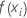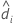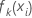# Fits and probabilities for Fit Model and Discover Key Predictors with TreeNet® Classification

###### Note

This command is available with the Predictive Analytics Module. Click here for more information about how to activate the module.

The expression of the relationship between the fits and the probabilities depends on the number of levels in the response variable:

## Binary response

The fitted value for the ith row with the optimal model is in terms of the odds:

where the odds have their customary definition:

The following relationship converts the fitted values into the probability for the event:
The probability for the non-event has the customary form:

## Multinomial response

The fitted value for the ith row with the optimal model has the following form:

The following relationship converts the fitted values into the probability for one of the outcomes, k.

For example, the probability for the outcome coded as 1 from a multinomial response with three levels has the following form:

## Notation

TermDescriptionfitted value for the ith rowfitted odds for the eventfitted value for the ith row for the kth level of the response variable
By using this site you agree to the use of cookies for analytics and personalized content.  Read our policy